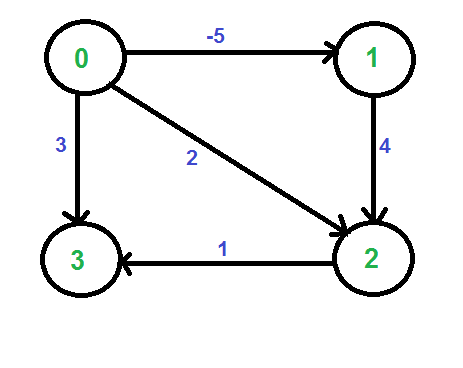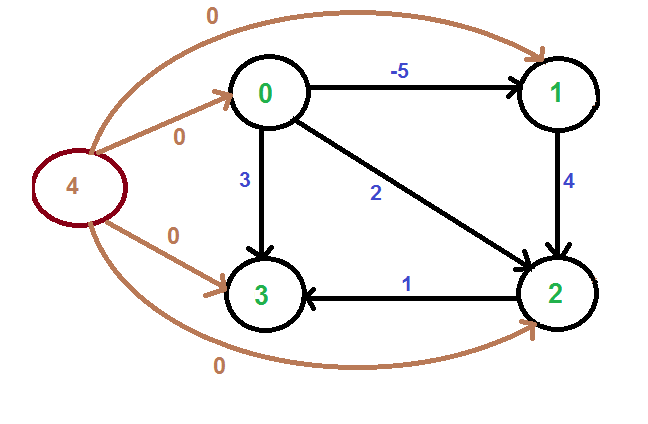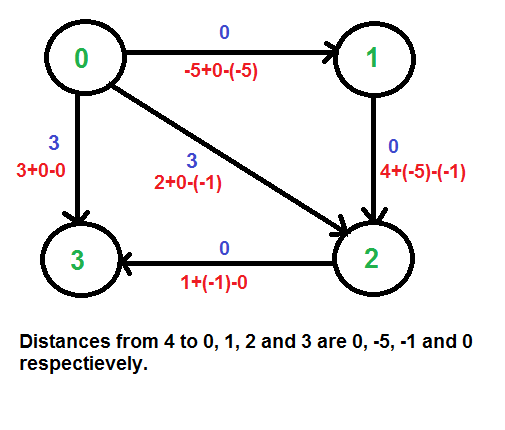Related Articles
Johnson’s algorithm for All-pairs shortest paths
• Difficulty Level : Hard
• Last Updated : 22 Mar, 2017

The problem is to find shortest paths between every pair of vertices in a given weighted directed Graph and weights may be negative. We have discussed Floyd Warshall Algorithm for this problem. Time complexity of Floyd Warshall Algorithm is Θ(V3). Using Johnson’s algorithm, we can find all pair shortest paths in O(V2log V + VE) time. Johnson’s algorithm uses both Dijkstra and Bellman-Ford as subroutines.

If we apply Dijkstra’s Single Source shortest path algorithm for every vertex, considering every vertex as source, we can find all pair shortest paths in O(V*VLogV) time. So using Dijkstra’s single source shortest path seems to be a better option than Floyd Warshell, but the problem with Dijkstra’s algorithm is, it doesn’t work for negative weight edge.
The idea of Johnson’s algorithm is to re-weight all edges and make them all positive, then apply Dijkstra’s algorithm for every vertex.

How to transform a given graph to a graph with all non-negative weight edges?
One may think of a simple approach of finding the minimum weight edge and adding this weight to all edges. Unfortunately, this doesn’t work as there may be different number of edges in different paths (See this for an example). If there are multiple paths from a vertex u to v, then all paths must be increased by same amount, so that the shortest path remains the shortest in the transformed graph.
The idea of Johnson’s algorithm is to assign a weight to every vertex. Let the weight assigned to vertex u be h[u]. We reweight edges using vertex weights. For example, for an edge (u, v) of weight w(u, v), the new weight becomes w(u, v) + h[u] – h[v]. The great thing about this reweighting is, all set of paths between any two vertices are increased by same amount and all negative weights become non-negative. Consider any path between two vertices s and t, weight of every path is increased by h[s] – h[t], all h[] values of vertices on path from s to t cancel each other.

How do we calculate h[] values? Bellman-Ford algorithm is used for this purpose. Following is the complete algorithm. A new vertex is added to the graph and connected to all existing vertices. The shortest distance values from new vertex to all existing vertices are h[] values.

Algorithm:
1) Let the given graph be G. Add a new vertex s to the graph, add edges from new vertex to all vertices of G. Let the modified graph be G’.

2) Run Bellman-Ford algorithm on G’ with s as source. Let the distances calculated by Bellman-Ford be h, h, .. h[V-1]. If we find a negative weight cycle, then return. Note that the negative weight cycle cannot be created by new vertex s as there is no edge to s. All edges are from s.

3) Reweight the edges of original graph. For each edge (u, v), assign the new weight as “original weight + h[u] – h[v]”.

4) Remove the added vertex s and run Dijkstra’s algorithm for every vertex.

How does the transformation ensure nonnegative weight edges?
The following property is always true about h[] values as they are shortest distances.

`   h[v] <= h[u] + w(u, v) `

The property simply means, shortest distance from s to v must be smaller than or equal to shortest distance from s to u plus weight of edge (u, v). The new weights are w(u, v) + h[u] - h[v]. The value of the new weights must be greater than or equal to zero because of the inequality "h[v] <= h[u] + w(u, v)". Example:
Let us consider the following graph.We add a source s and add edges from s to all vertices of the original graph. In the following diagram s is 4.We calculate the shortest distances from 4 to all other vertices using Bellman-Ford algorithm. The shortest distances from 4 to 0, 1, 2 and 3 are 0, -5, -1 and 0 respectively, i.e., h[] = {0, -5, -1, 0}. Once we get these distances, we remove the source vertex 4 and reweight the edges using following formula. w(u, v) = w(u, v) + h[u] - h[v].Since all weights are positive now, we can run Dijkstra's shortest path algorithm for every vertex as source.

Time Complexity: The main steps in algorithm are Bellman Ford Algorithm called once and Dijkstra called V times. Time complexity of Bellman Ford is O(VE) and time complexity of Dijkstra is O(VLogV). So overall time complexity is O(V2log V + VE).
The time complexity of Johnson's algorithm becomes same as Floyd Warshell when the graphs is complete (For a complete graph E = O(V2). But for sparse graphs, the algorithm performs much better than Floyd Warshell.